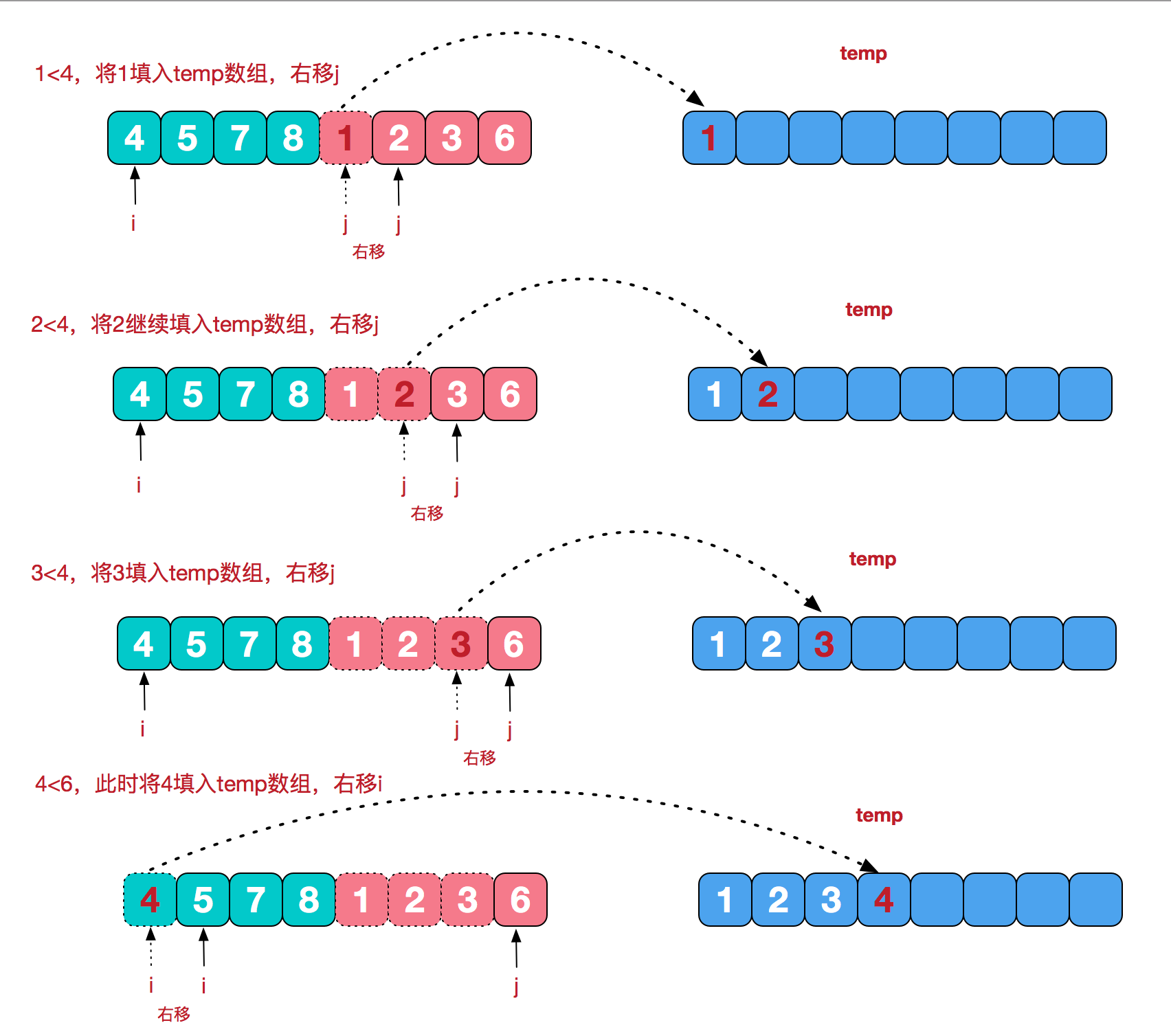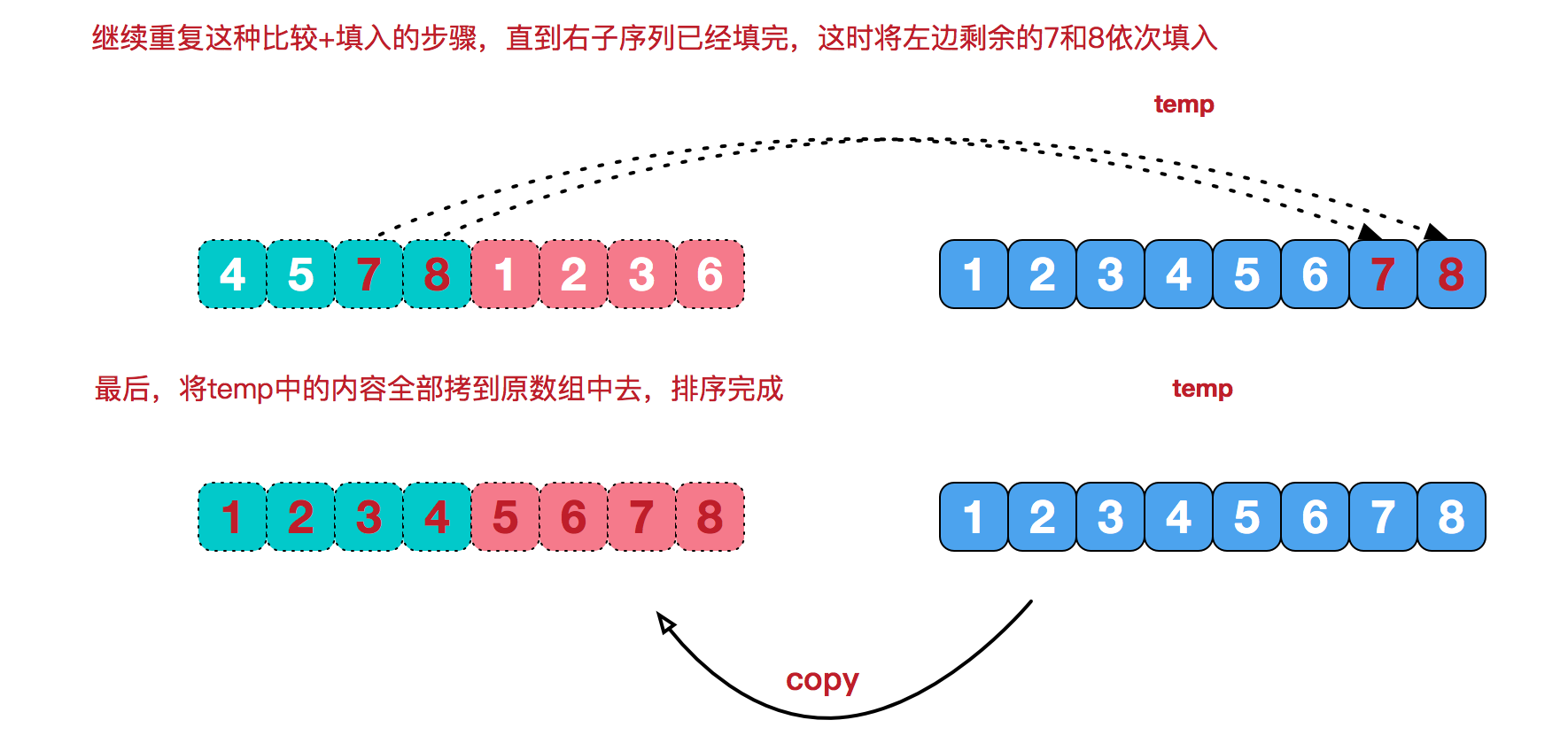# 合并相邻序列# 代码实现

```package com.ikonke.openapi;

import java.util.Arrays;

public class Test2 {
public static void main(String[] args) {
int[] arr = {9, 8, 7, 6, 5, 4, 3, 2, 1};
sort(arr);
System.out.println(Arrays.toString(arr));
}

/**
* 排序递归的方法
*
* @param arr
*/
public static void sort(int[] arr) {
/**
* 临时数组
*/
int[] temp = new int[arr.length];
sort(arr, 0, arr.length - 1, temp);
}

/**
* 分治的方法
*
* @param arr
* @param left
* @param right
* @param temp
*/
private static void sort(int[] arr, int left, int right, int[] temp) {
if (left < right) {
//从数组中间开始
int mid = (left + right) / 2;
//左边归并排序，使得左子序列有序
sort(arr, left, mid, temp);

//右边归并排序，使得右子序列有序
sort(arr, mid + 1, right, temp);

//将两个有序子数组合并操作
merge(arr, left, mid, right, temp);
}
}

/**
* 数组合并
*
* @param arr   原数组
* @param left
* @param mid
* @param right
* @param temp  临时数组
*/
private static void merge(int[] arr, int left, int mid, int right, int[] temp) {
int i = left;//左指针
int j = mid + 1;//右指针
int t = 0;//临时数组指针
while (i <= mid && j <= right) {
if (arr[i] <= arr[j]) {
temp[t++] = arr[i++];
} else {
temp[t++] = arr[j++];
}
}
while (i <= mid) {//将左边数组赋值到临时数组
temp[t++] = arr[i++];
}
while (j <= right) {//将右边数组赋值到临时数组
temp[t++] = arr[j++];
}
t = 0;
//数组拷贝替换
while (left <= right) {
arr[left++] = temp[t++];
}
}
}```

[1, 2, 3, 4, 5, 6, 7, 8, 9]